What is an allpass filter? What is it used for?

An allpass filter is a filter with a unity gain across all frequencies. This means that no frequency passing through that filter will be boosted or attenuated. It introduces, however, a frequency-dependent delay.

So although the output of an allpass filter doesn’t sound different from the input, this simple structure used in conjunction with other elements has an incredible power, which is present in almost all music software.

A digital allpass filter can be implemented as a finite-impulse response (FIR) filter or an infinite-impulse response (IIR) filter, typically of the first or the second order. Its applications are manifold: it is used to implement

• artificial reverberation,
• filters (e.g., highpass, lowpass, notch),
• audio effects (e.g., phaser),
• phase equalization, and more.

In this article, we will discuss the digital allpass filter in detail, present its various forms, provide their characteristics, schematics, and implementation, and, finally, discuss how you can use the allpass filter in your musical software like VST or AAX plugins.

##### In Short
###### Allpass Filter
• An allpass filter has gain equal to 1 at all frequencies.
• It delays all frequency components of the input, each by its own phase shift.
• It comes in various forms, but the most popular are first- and second-order IIR forms.
• It is a building block of a huge number of audio processing algorithms, like reverbs or filters.

1. Definition of an Allpass Filter
2. Types of Allpass Systems
1. FIR Allpass System
2. First-Order IIR Allpass
3. Second-Order IIR Allpass
4. Higher-Order IIR Allpass Filter
3. Applications of Allpass Filters
4. Example Allpass VST Plugin
5. Summary
6. Bibliography

## Definition of an Allpass Filter

An allpass filter is a filter which does not change the magnitude of any frequency component that passes through it .

Formally, if we denote the transfer function of an allpass filter by $H_\text{AP}(j\omega)$, we can write $|H_\text{AP}(j\omega)| = 1$. Here, $\omega = 2 \pi f / f_s$, where $f$ is a frequency in Hz and $f_s$ is the sampling rate in Hz.

But wait, since the magnitude does not change, what do we need allpass filters for? We need them, because they introduce a frequency-dependent phase delay. In other words, we are able to manipulate the phase of the frequency components without altering their magnitude.

Note: A transfer function of a digital filter is a Fourier transform or a $z$-transform of its impulse response. We denote them by $H(j\omega)$ and $H(z)$ respectively.

## Types of Allpass Systems

There are many types of systems that have the allpass property. They can be roughly divided into FIR and IIR categories.

### FIR Allpass System

The simplest allpass filter is the delay 

$H_\text{AP}(z) = \pm z^{-K}, \quad (1)$

where $K$ is an integer not smaller than 0, $K \geq 0$. Note that a unit delay $H_\text{AP}(z) = z^{-1}$ is also an allpass filter. Phase can be unaltered or inverted (multiplication by 1 or -1 respectively), because we are operating in the real domain.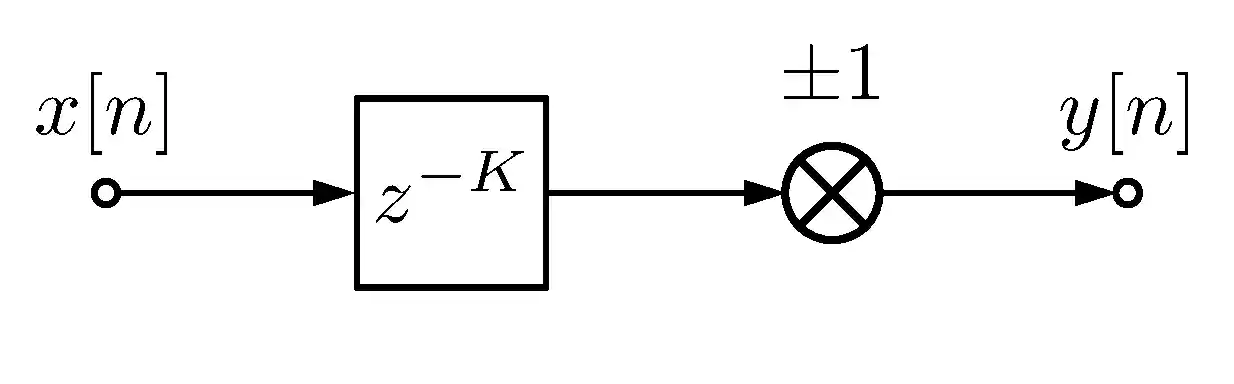Figure 1. Block diagram of an FIR allpass filter.

### First-Order IIR Allpass

A first-order IIR allpass filter is given by the following transfer function [2,3,4]

$H_{\text{AP}_1}(z) = \frac{a_1 + z^{-1}}{1 + a_1z^{-1}}, \quad (2)$

where $a \in \mathbb{R}$, because we consider real-valued filters only. The above equation corresponds to the following difference equation

$y[n] = a_1 x[n] + \underbrace{x[n - 1] - a_1 y[n-1]}_{d[n-1]}, \quad (3)$

where $x[n]$ is the input signal, $y[n]$ is the output signal, and $d[n-1]$ can be stored in a buffer as an intermediate value (a small implementation tip for you 😉).

#### Implementation

The difference equation 3 is equivalent to the following DSP diagram.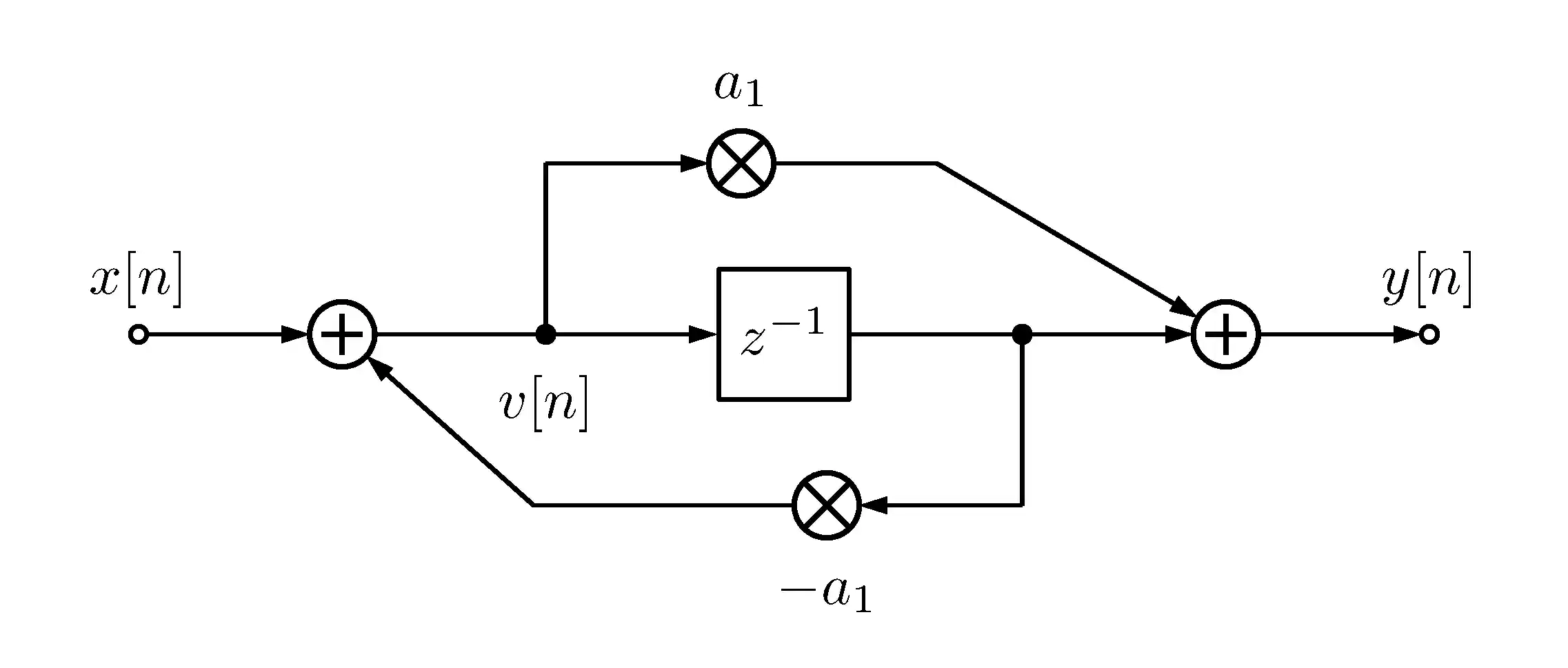Figure 2. Block diagram of the first-order allpass filter.

How to see the equivalence? First, observe that we have here a combination of two comb filters: a feedback and a feedforward comb filter .

Feedback comb filter difference equation:

$v[n] = x[n] - a_1 v[n-1]. \quad (4)$

Feedforward comb filter difference equation:

$y[n] = a_1 v[n] + v[n-1]. \quad (5)$

Second, insert $v[n]$ from Eq. 4 into Eq. 5:

$y[n] = a_1 x[n] - a_1^2 v[n-1] + v[n-1]. \quad (6)$

Finally, replace the first $v[n-1]$ with $\frac{y[n-1] - v[n-2]}{a_1}$ (from Eq. 5) and the second $v[n-1]$ with $x[n-1] - a_1 v[n-2]$ (from Eq. 4):

$y[n] = a_1 x[n] - a_1^2 \frac{y[n-1] - v[n-2]}{a_1} + x[n-1] - a_1 v[n-2] \\= a_1 x[n] - a_1 y[n-1] + x[n-1], \quad (7)$

which is equivalent to Eq. 3.

#### Magnitude Response

Why is this system allpass? Let’s calculate its magnitude transfer function at the unit circle, i.e., its magnitude frequency response or $\Bigl\lvert H_{\text{AP}_1}(z) \Bigr\rvert$ for $z = e^{j\omega}$.

$\Bigl\lvert H_{\text{AP}_1}(j\omega) \Bigr\rvert = \Bigl\lvert \frac{a_1 + e^{-j\omega}}{1 + a_1e^{-j\omega}} \Bigr\rvert = \Bigl\lvert e^{-j\omega} \frac{a_1e^{j\omega} + 1}{a_1e^{-j\omega} + 1} \Bigr\rvert = \bigl\lvert e^{-j\omega} \bigr\rvert \Bigl\lvert \frac{\overline{a_1 e^{-j\omega} + 1}}{ a_1 e^{-j\omega} + 1} \Bigr\rvert = 1, \quad (8)$

where $\overline{z}$ denotes the complex conjugate of $z$. We used the facts that $\Bigl\lvert e^{-j\omega} \Bigr\rvert = 1$ and $\Bigl\lvert \frac{\overline{z}}{z} \Bigr\rvert = 1$.

#### Phase Response

What is the role of the $a_1$ (allpass) coefficient? It controls the break frequency of the allpass filter. What is the break frequency? It is the frequency at which the phase shift of the first-order allpass filter is exactly $-\frac{\pi}{2}$ rad. To understand the break frequency we need to look at the phase frequency response of the allpass filter.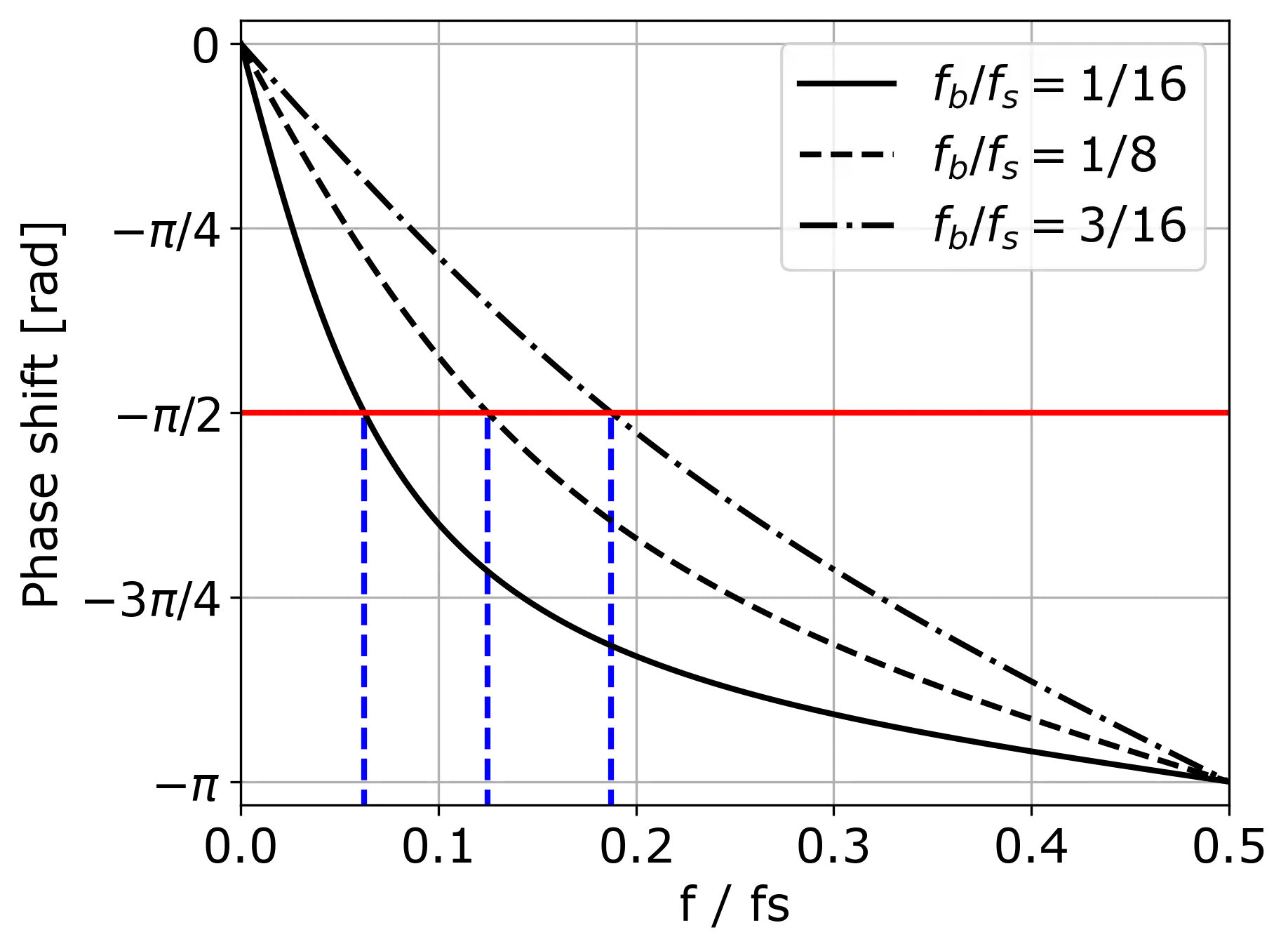Figure 3. Phase response of a first-order allpass filter for different break frequencies $f_\text{b}$.

We here refer to the digital frequency, i.e., the ratio of the frequency $f$ in Hz to the sampling rate $f_s$ in Hz.

The blue dashed lines in the figure mark the break frequencies of particular curves. The red line marks the phase shift by $-\frac{\pi}{2}$ rad. If we want to set a desired break frequency $f_\text{b}$ in radians, we can use the following formula to compute the $a_1$ coefficient [3,4,5]

$a_1 = \frac{\tan(\pi f_\text{b} / f_s) - 1}{\tan(\pi f_\text{b} / f_s) + 1}. \quad (9)$

How are Equations 2 and 9 derived? They are the result of transforming an analog allpass filter to the digital domain via the bilinear transform. Explaining this process is beyond the scope of this article; if you are interested, check out a great explanation in . After the derivations, we arrive exactly at the transfer function from Equation 2 with the coefficient $a_1$ given by Equation 9.

How are the plots in the above figure generated? They are derived from the argument (in the complex numbers sense) of the allpass transfer function $H_{\text{AP}_1}(j\omega)$. It can be done in software using the freqz function of Matlab or scipy.signal. Alternatively, you can use the following out-of-the-box formula 

$\text{Phase shift}(f) = - 2 \pi f / f_s + 2 \arctan \left( \frac{a_1 \sin (2 \pi f / f_s)}{1 + a_1 \cos (2 \pi f / f_s)} \right). \quad (10)$

#### Derive It Yourself?

Why am I saying that the formula in Equation 10 is out-of-the-box? I wasn’t able to derive it myself. After manual calculations, however, I managed to obtain a formula which was equivalent in terms of the plots. Comparison against freqz also yielded an identical result.

Do you know how to derive Equation 10? If so, please, let me know in the comments!

#### Properties of the First-Order Allpass Filter

When you look at the figure with the phase response of the first-order allpass filter, you can read out interesting properties.

• The phase shift at DC ($f = 0$) is 0.
• The phase shift at the Nyquist frequency ($f / f_s = 0.5$) is $-\pi$ rad and is always the maximum phase delay.
• The phase shift at the break frequency $f_\text{b}$ is $-\frac{\pi}{2}$ rad.

#### Cascading Allpass Filters

Arranging allpass filters in a series results in the summation of phase delays. We can therefore obtain a phase delay of $-N \pi$ at $f / f_s = 0.5$ by cascading $N$ first-order allpass filters. This principle underlies the phaser algorithm.

### Second-Order IIR Allpass

The second-order IIR allpass filter has the following transfer function :

$H_{\text{AP}_2}(z) = \frac{-c + d(1-c) z^{-1} + z^{-2}}{1 + d(1-c) z^{-1} - c z^{-2}}, \quad (11)$

where the parameter $d$ controls the break (center, cutoff) frequency of the filter $f_\text{b}$ (at which the phase shift is $-\pi$) and the parameter $c$ is computed from the parameter $BW$ which determines the bandwidth (the steepness of the slope of the phase transition around the break frequency). The relations between these parameters are specified by the equations

$c = \frac{\tan(\pi BW / f_s) - 1}{\tan(\pi BW / f_s) + 1}, \quad (12)$

$d = - \cos(2\pi f_\text{b} / f_s), \quad (13)$

where $f_s$ is the sampling rate. Parameters $BW$, $f_\text{b}$, and $f_s$ are given in Hz.

Note how $BW$ is coupled with $c$ but not with $d$ and $f_\text{b}$ is coupled with $d$ but not with $c$. This allows us to smoothly control our filter’s properties.

#### Phase Response

The phase response of the second-order allpass filter with different break frequencies $f_\text{b}$ looks as follows: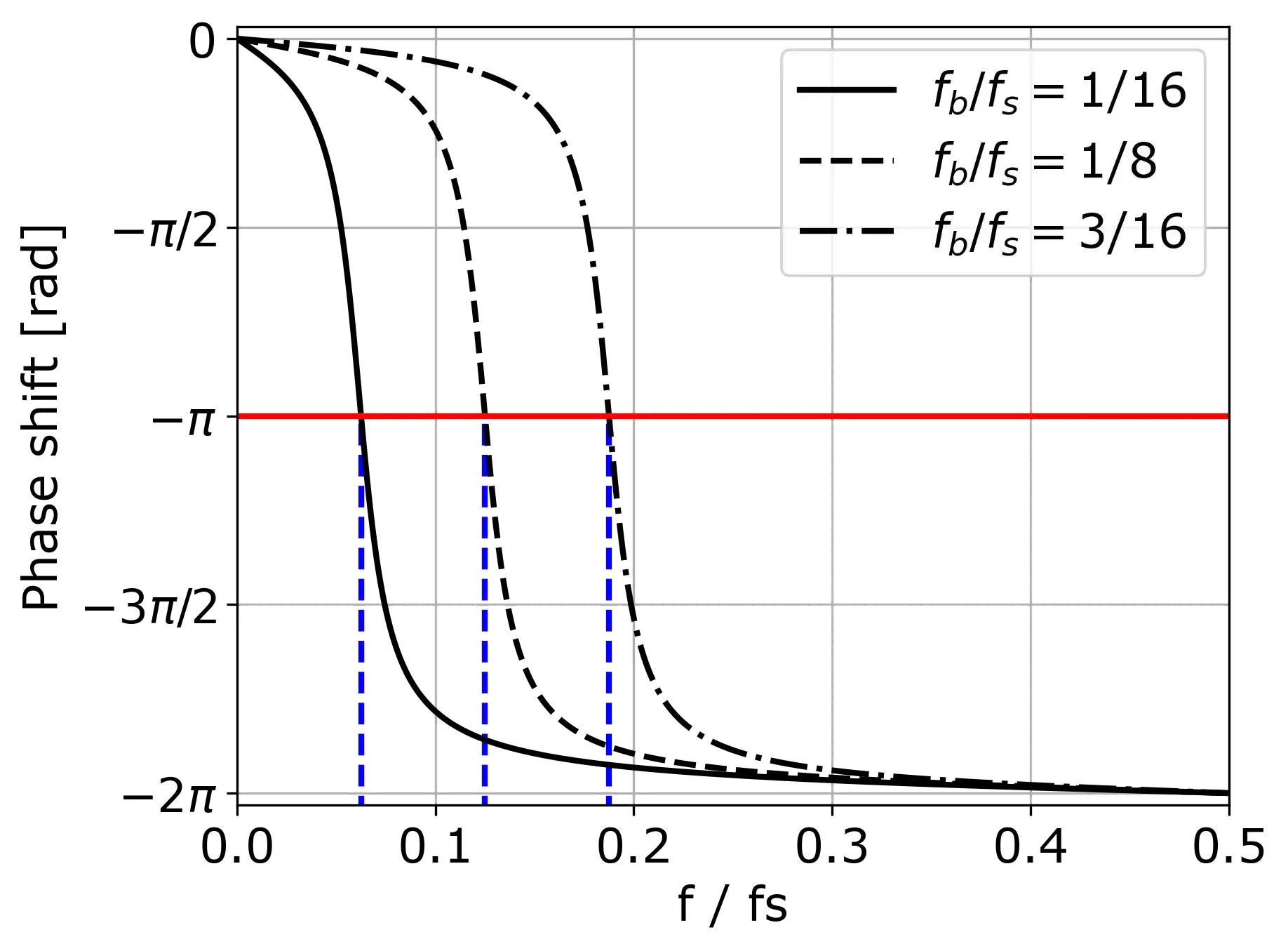Figure 4. Phase response of a second-order allpass filter for different break frequencies frequencies $f_\text{b}$ and bandwidth $BW / f_s = 0.022$.

As you can see above, the break frequency determines the point of the phase shift by $-\pi$. All slopes, however, have the same curvature.

If instead, we keep the break frequency constant and change the bandwidth parameter, we obtain the following phase responses: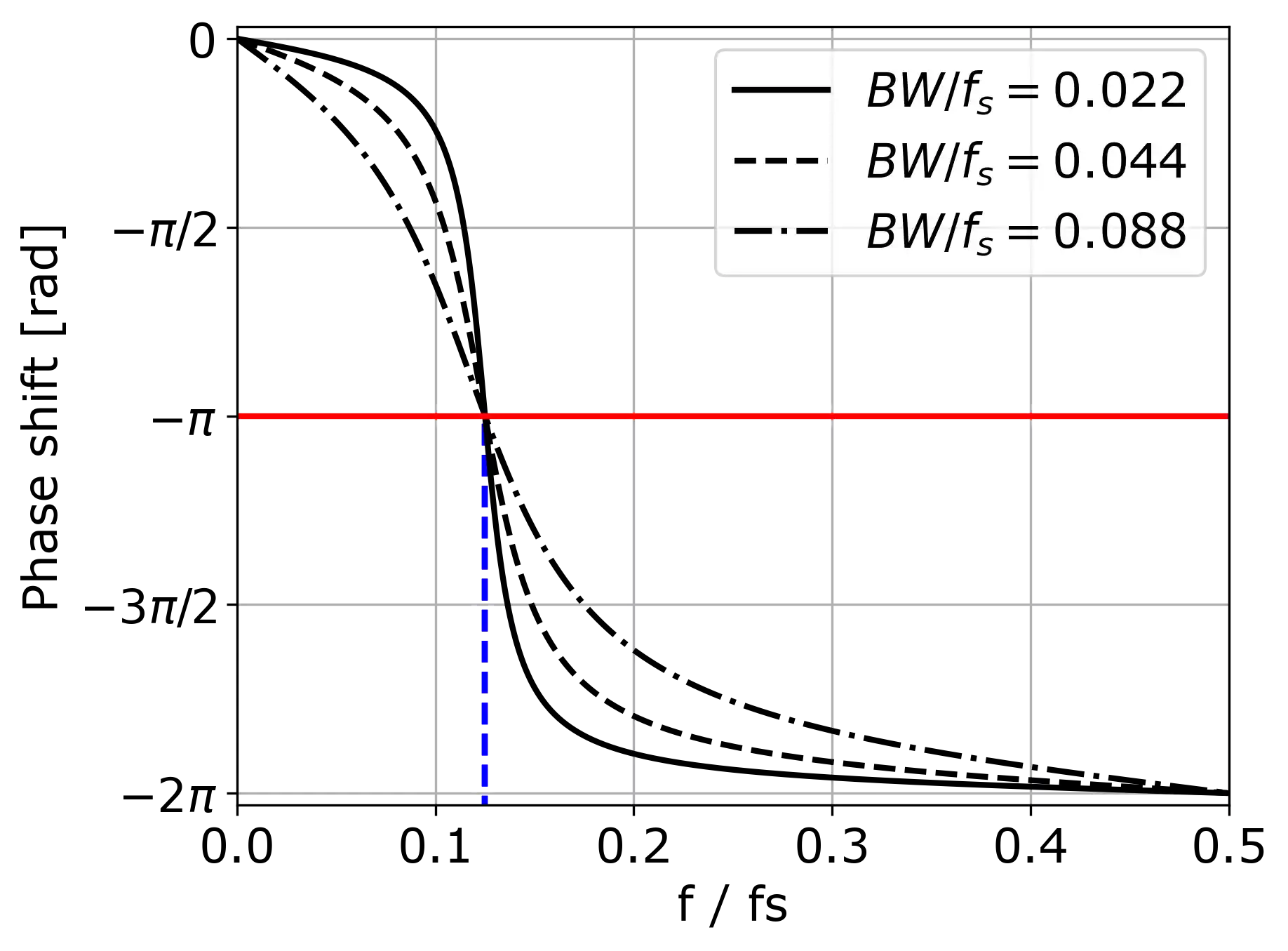Figure 5. Phase response of a second-order allpass filter for different bandwidths $BW$ and break frequency $f_\text{b} / f_s = 1/8$.

The curvature of the slope gets milder with the increasing $BW$ parameter but the $-\pi$ shift point remains at the same frequency.

What do these plots really show? They show that the second-order allpass filter is an incredibly flexible tool. We can independently change meaningful parameters such as the break frequency or the bandwidth while ensuring the filter’s stability. This property is crucial for implementing parametric filters (parametric equalizer, EQ), because we want to be able to change a filter’s properties in an intuitive and safe manner.

#### Implementation

The difference equation of the second-order allpass is 

$v[n] = x[n] - d(1 - c)v[n-1] + c v[n-2], \quad (14)$ $y[n] = -c v[n] + d (1-c) v[n-1] + v[n-2]. \quad (15)$

If that seems complicated, a diagram should make it clear 🙂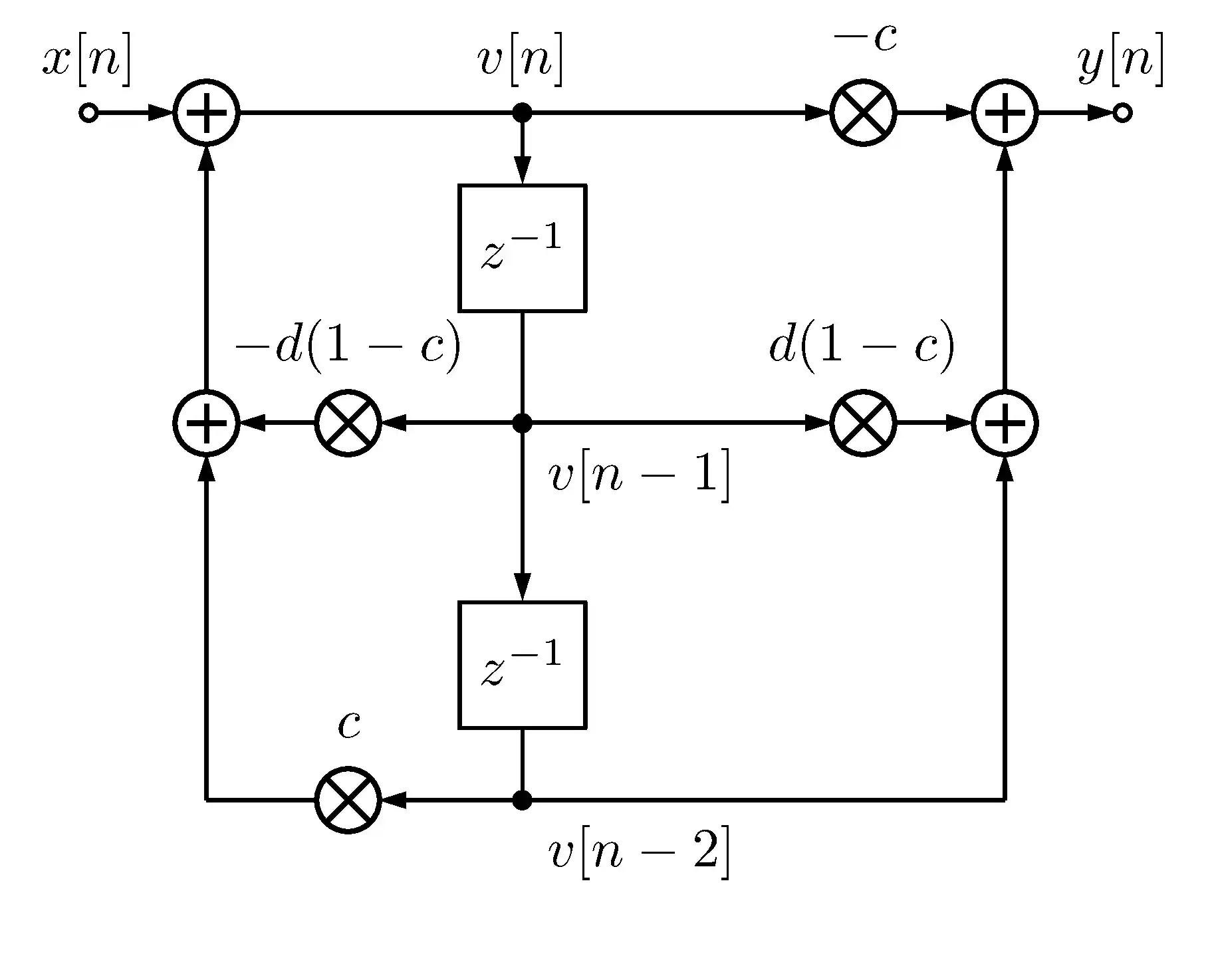Figure 6. Block diagram of the second-order allpass filter.

#### Properties of the Second-Order Allpass Filter

The second-order allpass filter has the following properties:

• The phase shift at DC ($f = 0$) is 0.
• The phase shift at the Nyquist frequency ($f / f_s = 0.5$) is $-2 \pi$ rad and is always the maximum phase delay.
• The phase shift at the break frequency $f_\text{b}$ is $-\pi$ rad.
• We can control the break frequency $f_\text{b}$ and the bandwidth $BW$ independently. This is not possible with the first-order allpass: there, the higher the break frequency, the less steep the slope.

### Higher-Order IIR Allpass Filter

You may have noticed a kind of symmetry in Equations 2 and 11. Indeed, it turns out that every IIR allpass filter must have a transfer function of the form 

$H_\text{AP} (z) = \pm z^{-K} \frac{\tilde{A}(z)}{A(z)}, \quad (16)$

where $K \in \mathbb{Z}$, $K \geq 0$, $A(z) = 1 + a_1 z^{-1} + a_2 z^{-2} + \dots + a_N z^{-N}$, and $\tilde{A}(z) = z^{-N} A(z^{-1})$. In other words, $\tilde{A}(z)$ is obtained by reversing the polynomial coefficients of $A(z)$.

Note that we added here a possible phase inversion and an additional delay. Thus, this formulation is 100% general and can be applied to every real-valued case.

However, higher-order allpass filters are rarely used in practice of audio programming, because their usage requires complicated analysis and, in most cases, first- and second-order IIR allpass filters suffice.

## Applications of Allpass Filters

Although for the single-channel audio, we cannot hear the effect of the phase delay, allpass filters are incredibly useful in musical applications. Why?

• They are stable.
• They have a meaningful parameters-to-coefficients mapping (e.g., break frequency to the $d$ coefficient in the second-order allpass).
• They are computationally efficient.
• Their properties are well-examined.

How can we use them in audio processing? For example, what happens if we add two sines at the same frequency: one delayed by $-\pi$ with respect to the other? They cancel out (destructive interference) and the output is zero.

Where can we use the properties of allpass filters? Below are some selected applications.

### Reverberation

Allpass filters are heavily used in artificial reverberation: an effect creating the impression of listening to music in some space (e.g., a room, a concert hall) . Allpass filters are present in some established approaches to simulate reverberation.

The Schroeder reverberator consists of a series of allpass filters, a parallel bank of feedback comb filters, and a mixing matrix . It was proposed as early as 1962!

The Freeverb algorithm uses a parallel bank of feedback comb filters and a series of allpass filters .

Note: If you are interested in how to implement the Freeverb using Rust, check out this video with Ian Hobson (ex-Ableton).

### Parametric Equalizer

Have you ever wondered, how are highpass, lowpass, shelving, notch or bandpass filters implemented in digital audio workstation (DAW) plugins? Well, I bet most of them use the RBJ Cookbook as a foundation. The RBJ Cookbook is a set of recipes for stable, controllable, and efficient filters of any type. It turns out, that all these recipes use first- or second- order allpass filters underneath! More details on how to derive these formulae can be found in … but I hope you will be able to read about them on WolfSound as well soon 🙂.

### Phaser

Do you know what’s the effect applied to guitars on Van Halen’s Eruption? That’s a phaser: an effect that sweeps notches through the spectrum of the input signal. One of the ways to implement a digital phaser is to use a chain of allpass filters, whose output is summed with the unfiltered input signal [3,4,5].

### Phase Equalization

Different microphones may introduce different frequency-dependent delays. Mixing their signals via summation could cause phase cancellation regardless of whether we invert the phase of one of the signals or not. We can prevent it only by selectively adjusting the phase delay of the frequency components of one of these signals. What is the system that can change the phase delay without changing the magnitude of a frequency component? I hope that you know the answer by now 🙂.

## Example Allpass VST Plugin

In Reaper’s ReaEQ VST plugin, there is an allpass filter available.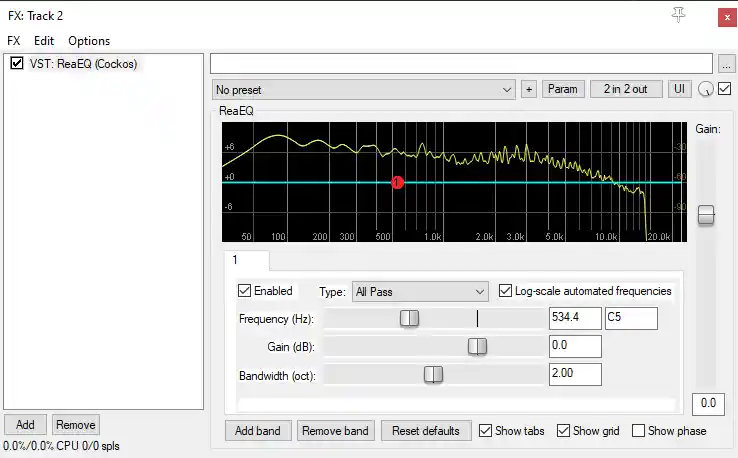Figure 7. Allpass filter in the Reaper’s ReaEQ VST plugin.

How to observe the frequency-dependent phase cancellation with a parallel allpass? Here’s a quick tutorial:Figure 8. Application of a parallel allpass filter with ReaEQ to create a notch.

Steps to reproduce:

1. Load an audio track to Reaper and duplicate it.
2. Add Reaper’s ReaEQ VST plugin to one of the tracks.
3. Open the plugin and remove all but 1 frequency bands.
4. Change the type of the remaining frequency band to “All Pass”.
5. While listening to the master track, change the “Frequency [Hz]” parameter of the allpass. Can you hear the notch in the frequency spectrum?
6. You can also load ReaEQ on the master track and observe the notch there.

Have fun! 🎧

## Summary

In this article, we have discussed an allpass filter. Now, you understand

• what is an allpass filter,
• what types of musically useful allpass filters exist,
• how to implement them,
• how they are applied in various effects,
• how to use an allpass filter in a DAW.

Thank you for reading! If you enjoyed the article and want to learn even more, sign up for my newsletter! You will become an expert in digital audio effects without the need to read thick books on DSP.

If you have any questions, don’t hesitate to ask them below!

## Bibliography

Links above may be affiliate links. That means that I may earn a commission if you decide to make a purchase. This does not incur any cost for you. Thank you.